Tamil Nadu Board of Secondary EducationHSC Science Class 11th

# Tamil Nadu Board Samacheer Kalvi solutions for Class 11th Physics Volume 1 and 2 Answers Guide chapter 2 - Kinematics [Latest edition]

#### Chapters## Chapter 2: Kinematics

Evaluation
Evaluation [Pages 98 - 102]

### Tamil Nadu Board Samacheer Kalvi solutions for Class 11th Physics Volume 1 and 2 Answers Guide Chapter 2 Kinematics Evaluation [Pages 98 - 102]

#### Multiple Choice Questions

Evaluation | Q I. 01. | Page 98

Which one of the following Cartesian coordinate systems are not followed in physics?

•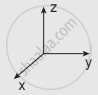•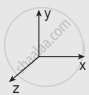•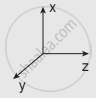•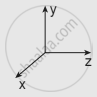Evaluation | Q I. 02. | Page 98

Identify the unit vector in the following.

• hati + hatj

• hati/sqrt2

• hatk - hatj/sqrt2

• (hati + hatj)/sqrt2

Evaluation | Q I. 03. | Page 98

Which one of the following physical quantities cannot be represented by a scalar?

• Mass

• length

• momentum

• magnitude of acceleration

Evaluation | Q I. 04. | Page 98

Two objects of masses m1 and m2 fall from the heights h1 and h2 respectively. The ratio of the magnitude of their momenta when they hit the ground is ______

• sqrt(h_1/h_2)

• sqrt((m_1h_1)/(m_2h_2))

• m_1/m_2sqrt(h_1/h_2)

• m_1/m_2

Evaluation | Q I. 05. | Page 98

If a particle has negative velocity and negative acceleration, its speed ______

• increases

• decreases

• remains same

• zero

Evaluation | Q I. 06. | Page 98

If the velocity is vecv = 2hati + t^2hatj - 9veck, then the magnitude of acceleration at t = 0.5 s is ______

• 1m s-2

• 2 m s-2

• zero

• -1 m s-2

Evaluation | Q I. 07. | Page 98

If an object is dropped from the top of a building and it reaches the ground at t = 4 s, then the height of the building is ______

(ignoring air resistance) (g = 9.8 ms–2)

• 77.3 m

• 78.4 m

• 80.5 m

• 79.2 m

Evaluation | Q I. 08. | Page 98

A ball is projected vertically upwards with a velocity v. It comes back to the ground in time t. Which v-t graph shows the motion correctly?

•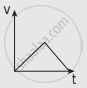•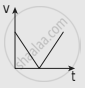•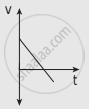•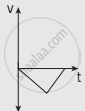Evaluation | Q I. 09. | Page 98

If one object is dropped vertically downward and another object is thrown horizontally from the same height, then the ratio of vertical distance covered by both objects at any instant t is ______

• 1

• 2

• 4

• 0.5

Evaluation | Q I. 10. | Page 99

A ball is dropped from some height towards the ground. Which one of the following represents the correct motion of the ball?

•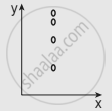•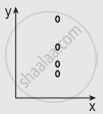•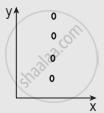•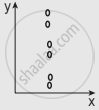Evaluation | Q I. 11. | Page 99

If a particle executes uniform circular motion in the xy plane in a clockwise direction, then the angular velocity is in ______

• +y direction

• +z direction

• -z direction

• -x direction

Evaluation | Q I. 12. | Page 99

If a particle executes uniform circular motion, choose the correct statement.

• The velocity and speed are constant.

• The acceleration and speed are constant.

• The velocity and acceleration are constant.

• The speed and magnitude of acceleration are constant.

Evaluation | Q I. 13. | Page 99

If an object is thrown vertically up with the initial speed u from the ground, then the time taken by the object to return back to the ground is ______

• u^2/(2g)

• u^2/(g)

• u/(2g)

• (2u)/g

Evaluation | Q I. 14. | Page 99

Two objects are projected at angles 30° and 60° respectively with respect to the horizontal direction. The range of two objects is denoted as R30° and R60°. Choose the correct relation from the following.

• R30° = R60°

• R30° = 4R60°

• R30° = R_{60°}/2

• R30° = 2R60°

Evaluation | Q I. 15. | Page 99

An object is dropped in an unknown planet from a height of 50 m, it reaches the ground in 2 s. The acceleration due to gravity in this unknown planet is ______

• g = 20 m s-2

• g = 25 m s-2

• g = 15 m s-2

• g = 30 m s-2

Evaluation | Q II. 1. | Page 99

Explain what is meant by Cartesian coordinate system?

Evaluation | Q II. 2. | Page 99

Define a vector. Give examples?

Evaluation | Q II. 3. | Page 99

Define a scalar. Give examples?

Evaluation | Q II. 4. | Page 99

Write a short note on the scalar product between two vectors.

Evaluation | Q II. 5. | Page 100

Write a short note on the vector product between two vectors.

Evaluation | Q II. 6. | Page 100

How do you deduce that two vectors are perpendicular?

Evaluation | Q II. 7. a. | Page 100

Define displacement.

Evaluation | Q II. 7. b. | Page 100

Define distance.

Evaluation | Q II. 8. a. | Page 100

Define velocity.

Evaluation | Q II. 8. b. | Page 100

Define speed.

Evaluation | Q II. 9. | Page 100

Define acceleration.

Evaluation | Q II. 10. | Page 100

What is the difference between velocity and average velocity?

Evaluation | Q II. 11. | Page 100

Evaluation | Q II. 12. a. | Page 100

Define angular displacement.

Evaluation | Q II. 12. b. | Page 100

Define angular velocity.

Evaluation | Q II. 13. | Page 100

What is non-uniform circular motion?

Evaluation | Q II. 14. | Page 100

Write down the kinematic equations for angular motion.

Evaluation | Q II. 15. | Page 100

Write down the expression for angle made by resultant acceleration and radius vector in the non-uniform circular motion.

Evaluation | Q III. 1. | Page 100

Explain in detail the triangle law of addition.

Evaluation | Q III. 2. | Page 100

Discuss the properties of scalar and vector products.

Evaluation | Q III. 3. | Page 100

Derive the kinematic equations of motion for constant acceleration.

Evaluation | Q III. 4. | Page 100

Derive the equations of motion for a particle (a) falling vertically (b) projected vertically.

Evaluation | Q III. 5. | Page 100

Derive the equation of motion, range, and maximum height reached by the particle thrown at an oblique angle θ with respect to the horizontal direction.

Evaluation | Q III. 6. | Page 100

Derive the expression for centripetal acceleration.

Evaluation | Q III. 7. | Page 100

Derive the expression for total acceleration in the non-uniform circular motion.

Evaluation | Q IV. 1. | Page 100

The position vector particle has a length of 1m and makes 30° with the x-axis. What are the lengths of the x and y components of the position vector?

Evaluation | Q IV. 2. | Page 100

A particle has its position moved from vecr_1 =  3hati + 4hatj to vecr_2 =  hati + 2hatj. Calculate the displacement vector (∆vecr) and draw the vecr_1,  vecr_2 and Deltavecr vector in a two dimensional Cartesian coordinate system.

Evaluation | Q IV. 3. | Page 100

Calculate the average velocity of the particle whose position vector changes from vecr_1 = 5hati + 6hatj  to vecr_2 = 2hati + 3hatj in a time 5 second.

Evaluation | Q IV. 4. | Page 100

Convert the vector vecr = 3hati + 2hatj into a unit vector.

Evaluation | Q IV. 5. | Page 100

What are the resultants of the vector product of two given vectors given by vecA = 4hati - 2hatj + hatk and vecB = 5hati + 3hatj - 4hatk?

Evaluation | Q IV. 6. | Page 101

An object at an angle such that the horizontal range is 4 times the maximum height. What is the angle of projection of the object?

Evaluation | Q IV. 7. | Page 101

The following graphs represent the velocity-time graph. Identify what kind of motion a particle undergoes in each graph.

 (a)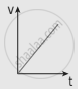(b)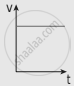(c)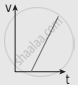(d)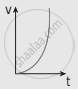Evaluation | Q IV. 8. | Page 101

The following velocity-time graph represents a particle moving in the positive x-direction. Analyze its motion from 0 to 7 s. Calculate the displacement covered and distance travelled by the particle from 0 to 2 s.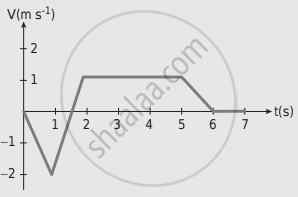Evaluation | Q IV. 9. | Page 101

A particle is projected at an angle of θ with respect to the horizontal direction. Match the following for the above motion.

(a) vx – decreases and increases
(b) vy – remains constant
(c) Acceleration – varies
(d) Position vector – remains downward

Evaluation | Q IV. 10. | Page 101

A water fountain on the ground sprinkles water all around it. If the speed of the water coming out of the fountain is v. Calculate the total area around the fountain that gets wet.

Evaluation | Q IV. 11. | Page 101

The following table gives the range of a particle when thrown on different planets. All the particles are thrown at the same angle with the horizontal and with the same initial speed. Arrange the planets in ascending order according to their acceleration due to gravity, (g value).

 Planet Range Jupiter 50 m Earth 75 m Mars 90 m Mercury 95m

Evaluation | Q IV. 12. | Page 101

The resultant of two vectors A and B is perpendicular to vector A and its magnitude is equal to half of the magnitude of vector B. Then the angle between A and B is

• 30°

• 45°

• 150°

• 120°

Evaluation | Q IV. 13. | Page 101

Compare the components for the following vector equations

(a) "T"hatj - "mg"hatj = "ma"hatj
(b) vecT + vecF = vecA + vecB
(c) vecT - vecF = vecA - vecB
(d) "T"hatj + "mg"hatj = "ma"hatj

Evaluation | Q IV. 14. | Page 102

Calculate the area of the triangle for which two of its sides are given by the vectors vecA = 5hati - 3hatj, vecB = 4hati + 6hatj

Evaluation | Q IV. 15. | Page 102

If Earth completes one revolution in 24 hours, what is the angular displacement made by Earth in one hour? Express your answer in both radian and degree.

Evaluation | Q IV. 16. | Page 102

An object is thrown with an initial speed of 5 m s-1 with an angle of projection of 30°. What is the height and range reached by the particle?

Evaluation | Q IV. 17. | Page 102

A football player hits the ball with a speed of 20 m s-1 with an angle of 30° with respect to horizontal direction as shown in the figure. The goal post is at a distance of 40 m from him. Find out whether the ball reaches the goal post?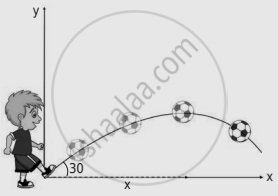Evaluation | Q IV. 18. | Page 102

If an object is thrown horizontally with an initial speed of 10 ms -1 from the top of a building of height 100 m. What is the horizontal distance covered by the particle?

Evaluation | Q IV. 19. | Page 102

An object is executing uniform circular motion with an angular speed of pi/12 radians per second. At t = 0 the object starts at an angle θ = 0 What is the angular displacement of the particle after 4 s?

Evaluation | Q IV. 20. | Page 102

Consider the x-axis as representing east, the y–axis as north, and the z-axis as vertically upwards. Give the vector representing each of the following points.

a) 5 m northeast and 2 m up

b) 4 m southeast and 3 m up

c) 2 m northwest and 4 m up

Evaluation | Q IV. 21. | Page 102

The Moon is orbiting the Earth approximately once in 27 days, what is the angle transversed by the Moon per day?

Evaluation | Q IV. 22. | Page 102

An object of mass m has angular acceleration α = 0.2 rad s-2. What is the angular displacement covered by the object after 3 seconds? (Assume that the object started with angle zero with zero angular velocity).

## Chapter 2: Kinematics

Evaluation## Tamil Nadu Board Samacheer Kalvi solutions for Class 11th Physics Volume 1 and 2 Answers Guide chapter 2 - Kinematics

Tamil Nadu Board Samacheer Kalvi solutions for Class 11th Physics Volume 1 and 2 Answers Guide chapter 2 (Kinematics) include all questions with solution and detail explanation. This will clear students doubts about any question and improve application skills while preparing for board exams. The detailed, step-by-step solutions will help you understand the concepts better and clear your confusions, if any. Shaalaa.com has the Tamil Nadu Board of Secondary Education Class 11th Physics Volume 1 and 2 Answers Guide solutions in a manner that help students grasp basic concepts better and faster.

Further, we at Shaalaa.com provide such solutions so that students can prepare for written exams. Tamil Nadu Board Samacheer Kalvi textbook solutions can be a core help for self-study and acts as a perfect self-help guidance for students.

Concepts covered in Class 11th Physics Volume 1 and 2 Answers Guide chapter 2 Kinematics are Introduction to Kinematics, Concept of Rest and Motion, Elementary Concept of Vector Algebra, Multiplication of Vector by a Scalar, Position Vectors, Distance and Displacement, Differential Calculus, Integral Calculus, Motion Along One Dimension, Projectile Motion, Components of Vector.

Using Tamil Nadu Board Samacheer Kalvi Class 11th solutions Kinematics exercise by students are an easy way to prepare for the exams, as they involve solutions arranged chapter-wise also page wise. The questions involved in Tamil Nadu Board Samacheer Kalvi Solutions are important questions that can be asked in the final exam. Maximum students of Tamil Nadu Board of Secondary Education Class 11th prefer Tamil Nadu Board Samacheer Kalvi Textbook Solutions to score more in exam.

Get the free view of chapter 2 Kinematics Class 11th extra questions for Class 11th Physics Volume 1 and 2 Answers Guide and can use Shaalaa.com to keep it handy for your exam preparation